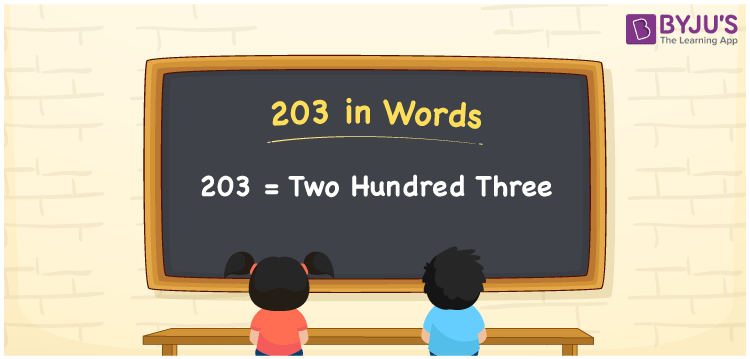# 203 in Words

We can write 203 in words as Two hundred three. That means the number name for 203 is Two hundred three”. Generally, number names are used to express or convey the given numbers to others. Suppose you spent Rs. 203 for breakfast in a day, then you can say, “I spent Two hundred three rupees for breakfast”. Learn how to convert the cardinal number 203 into words here in this article.

 203 in words Two hundred three Two hundred three in Numbers 203

## 203 in English Words

We generally write numbers in words using the English alphabet. Thus, we can spell 203 in English words as “Two hundred three”.## How to Write 203 in Words?

Let us create a three-column place value chart to convert the number 203 into words since it is a three-digit number. This can be done as follows.

 Hundreds Tens Ones 2 0 3

Here, ones = 3, tens = 0, hundreds = 2

2 × Hundred + 0 × Ten + 3 × One

= 2 × 100 + 3 × 1

= 200 + 3

= Two hundred + Three

= Two hundred three

Therefore, 203 in words = Two hundred three

203 is a natural number that precedes 204 and succeeds 202.

203 in words – Two hundred three

Is 203 an odd number? – Yes

Is 203 an even number? – No

Is 203 a prime number? – No

Is 203 a composite number? – Yes

Is 203 a perfect square number? – No

Is 203 a perfect cube number? – No

## Frequently Asked Questions on 203 in Words

Q1

### How do you write 203 in words?

The numeral 203 can be written in words as “Two hundred three”.
Q2

### Express 0.203 in word form.

We can express the decimal number 0.203 in word form as “Zero point two zero three”.
Q3

### Write 10^203 in words.

We can write the expression 10^203 in words as “Ten to the power of two hundred three”.